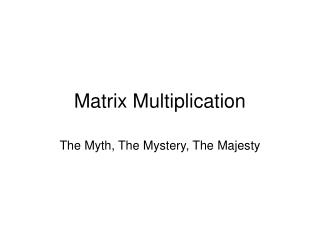DownloadDownload PresentationMatrix Multiplication

# Matrix Multiplication

Download Presentation## Matrix Multiplication

- - - - - - - - - - - - - - - - - - - - - - - - - - - E N D - - - - - - - - - - - - - - - - - - - - - - - - - - -
##### Presentation Transcript

1. Matrix Multiplication The Myth, The Mystery, The Majesty

2. Matrix Multiplication • Simple, yet important problem • Many fast serial implementations exist • Atlas • Vendor BLAS • Natural to want to parallelize

3. The Problem • Take two matrices, A and B, and multiply them to get a 3rd matrix C • C = A*B (C = B*A is a different matrix) • C(i,j) = vector product of row i of A with column j of B • The “classic” implementation uses the triply nested loop • for (i…) • for (j …) • for (k…) • C[i][j] += A[i][k] * B[k][j]

4. Parallelizing Matrix Multiplication • The problem can be parallelized in many ways • Simplest is to replicate A and B on every processor and break up the iteration space by rows • for (i = lowerbound; i < upperbound; i++) … • This method is easy to code and has good speedups, but very poor memory scalability

5. Parallelizing MM • One can refine the previous approach by only storing the rows of A that each processor needs • Better, but still needs all of B • 1-D Partitioning is simple to code, but has very poor memory scalability

6. Parallelizing MM • 2-D Partitioning • Instead break up the matrix into blocks • Each processor stores a block of C to compute and “owns” a block of A and a block of B • Now one only needs to know about the other blocks of A in the same rows and the blocks of B in the same columns • One can buffer all necessary blocks or only one block of A and B at a time with some smart tricks (Fox’s Algorithm / Cannon’s Algorithm) • Harder to code, but memory scalability is MUCH better • Bonus - If the blocks become sufficiently small, can exploit good cache behavior

7. OpenMP • 1-D partitioning easy to code – parallel for • Unclear what data lives where • 2-D partitioning possible – each processor needs to compute its bounds and work on them in a parallel section • Again, not sure what data lives where so no guarantees this helps 1024x1024 Matrix

8. MPI • 1-D very easy to program with data replication • 2-D relatively simple to program as well • Only requires two broadcasts • Send A block to my row • Send B block to my column • Fancier algorithms replace broadcasts with circular shifts so that the right blocks arrive at the right time

9. Others • HPF and Co-array Fortran both let you specify data distribution • One could conceivably have either 1-D or 2-D versions with good performance • Charm • I suppose you could do either 1-D or 2-D versions, but MM is a very regular problem so none of the load-balancing/etc. is needed. Easier to just use MPI…. • STAPL • Wrong paradigm.

10. Distributed vs Shared Memory? • Neither is necessarily better • The problem handles parallelization better if the distribution of data in memory is clearly defined